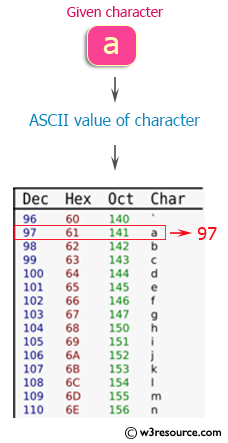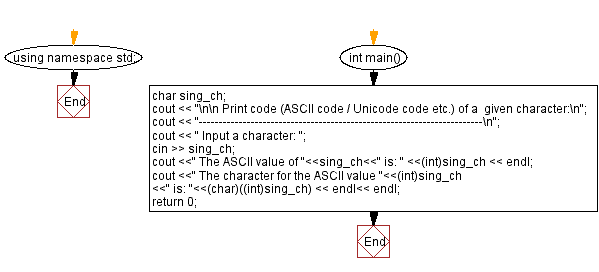﻿ C++ Exercises: Print the code of a given character - w3resource

# C++ Exercises: Print the code of a given character

## C++ Basic: Exercise-49 with Solution

Write a C++ program to print the code (ASCII code / Unicode code etc.) of a given character.

Pictorial Presentation:Sample Solution:

C++ Code :

``````#include <iostream>
using namespace std;
int main()
{
char sing_ch;
cout << "\n\n Print code (ASCII code / Unicode code etc.) of a  given character:\n";
cout << "-----------------------------------------------------------------------\n";
cout << " Input a character: ";
cin >> sing_ch;

cout <<" The ASCII value of "<<sing_ch<<" is: " <<(int)sing_ch << endl;
cout <<" The character for the ASCII value "<<(int)sing_ch <<" is: "<<(char)((int)sing_ch) << endl<< endl;
return 0;
}
``````

Sample Output:

``` Print code (ASCII code / Unicode code etc.) of a  given character:
-----------------------------------------------------------------------
Input a character: a
The ASCII value of a is: 97
The character for the ASCII value 97 is: a
```

Flowchart:C++ Code Editor:

What is the difficulty level of this exercise?

﻿

## C++ Programming: Tips of the Day

Why is there no std::stou?

The most pat answer would be that the C library has no corresponding "strtou", and the C++11 string functions are all just thinly veiled wrappers around the C library functions: The std::sto* functions mirror strto*, and the std::to_string functions use sprintf.

Ref: https://bit.ly/3wtz2qA

We are closing our Disqus commenting system for some maintenanace issues. You may write to us at reach[at]yahoo[dot]com or visit us at Facebook